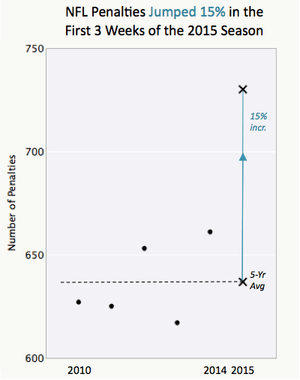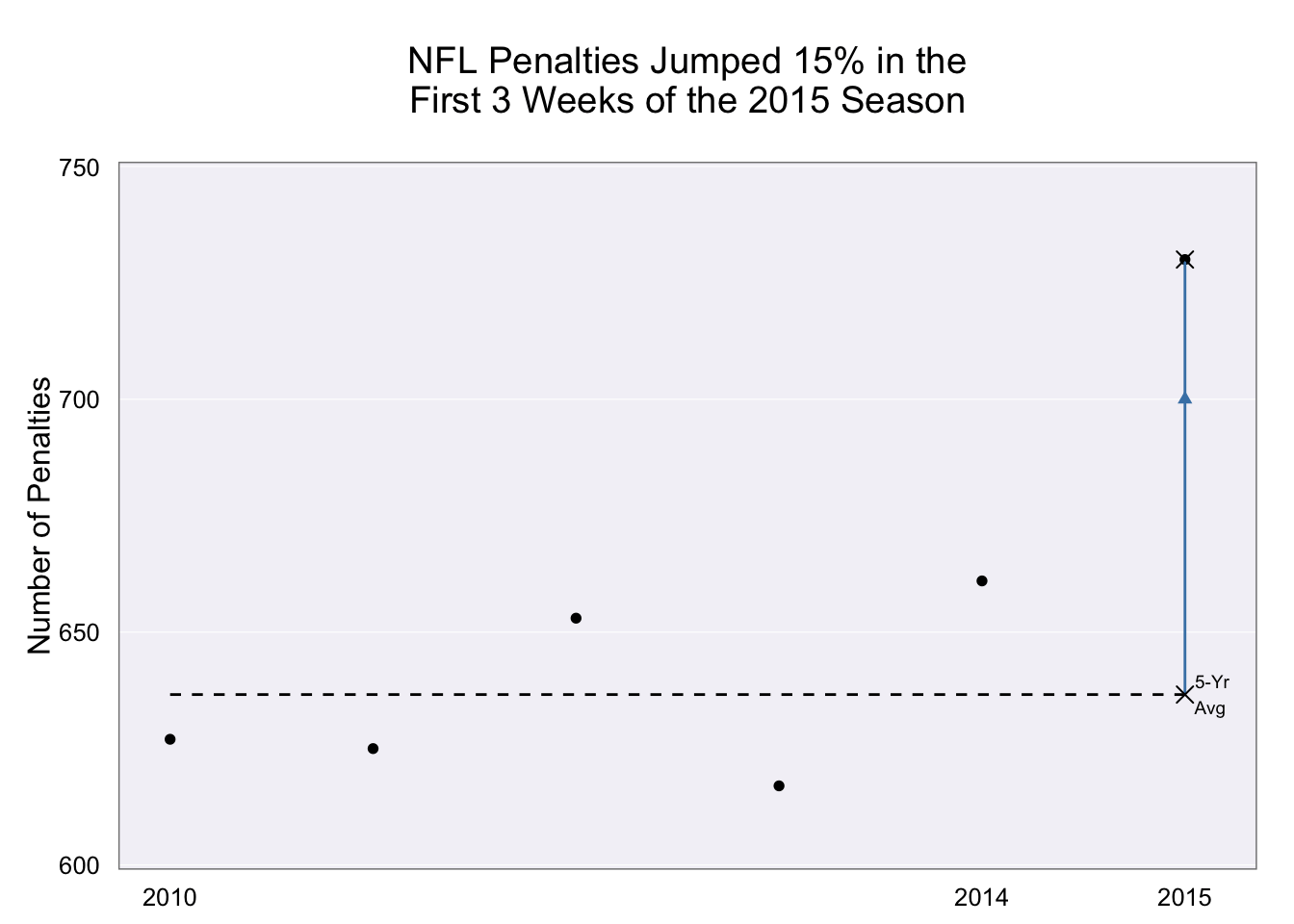[This article was first published on rud.is » R, and kindly contributed to R-bloggers]. (You can report issue about the content on this page here)
Want to share your content on R-bloggers? click here if you have a blog, or here if you don't.

Junk Charts did a post on Don’t pick your tool before having your design and made a claim that this:“cannot be produced directly from a tool (without contorting your body in various painful locations)”.

I beg to differ.

With R & ggplot2, I get to both pick my tool and design at the same time since I have a very flexible and multi-purpose tool. I also don’t believe that the code below qualifies as “contortions”, though I am a ggplot2 fanboi. It’s no different than Excel folks clicking on radio buttons and color pickers, except my process is easily repeatable & scalable once finalized (this is not finalized as it’s not 100% parameterized but it’s not difficult to do that last part).

```library(ggplot2)   dat <- data.frame(year=2010:2015, penalties=c(627, 625, 653, 617, 661, 730))   avg <- data.frame(val=mean(head(dat\$penalties, -1)), last=dat\$penalties, lab="5-YrnAvg")   gg <- ggplot(dat, aes(x=year, y=penalties)) gg <- gg + geom_point() gg <- gg + scale_x_continuous(breaks=c(2010, 2014, 2015), limits=c(NA, 2015.1)) gg <- gg + scale_y_continuous(breaks=c(600, 650, 700, 750), limits=c(599, 751), expand=c(0,0)) gg <- gg + geom_segment(data=avg, aes(x=2010, xend=2015, y=val, yend=val), linetype="dashed") gg <- gg + geom_segment(data=avg, aes(x=2015, xend=2015, y=val, yend=last), color="steelblue") gg <- gg + geom_point(data=avg, aes(x=2015, y=val), shape=4, size=3) gg <- gg + geom_text(data=avg, aes(x=2015, y=val), label="5-YrnAvg", size=2.5, hjust=-0.3) gg <- gg + geom_point(data=avg, aes(x=2015, y=700), shape=17, col="steelblue") gg <- gg + geom_point(data=avg, aes(x=2015, y=730), shape=4, size=3) gg <- gg + labs(x=NULL, y="Number of Penalties", title="NFL Penalties Jumped 15% in thenFirst 3 Weeks of the 2015 Seasonn") gg <- gg + theme_bw() gg <- gg + theme(panel.grid.minor=element_blank()) gg <- gg + theme(panel.grid.major.x=element_blank()) gg <- gg + theme(panel.grid.major.y=element_line(color="white")) gg <- gg + theme(panel.background=element_rect(fill="#f3f2f7")) gg <- gg + theme(axis.ticks=element_blank()) gg```